This lesson is being piloted (Beta version)

# Raster Calculations in R

## Overview

Teaching: 40 min
Exercises: 20 min
Questions
• How do I subtract one raster from another and extract pixel values for defined locations?

Objectives
• Perform a subtraction between two rasters using raster math.

• Perform a more efficient subtraction between two rasters using the raster `overlay()` function.

• Export raster data as a GeoTIFF file.

##The Data

In this lesson, we will be working with two field sites: the Harvard Forest (`HARV`) and San Joaquin Experimental Range (`SJER`).

In this lesson, the rasters we will use are: `HARV_dsmCrop.tif` (DSM) and `HARV_dtmCrop.tif` (DTM) from `HARV`. We will also use `SJER_dsmCrop.tif` (DSM) and `SJER_dtmCrop.tif` (DTM) from `SJER`.

If you are starting this lesson from the beginning, you will need to create dataframes from each of these four rasters using `as.data.frame()` and specifying the argument `xy=TRUE`.

If you are continuing this lesson from episode 03, you should have these objects and dataframes already in your `R` environment.

``````# Learners will have these data loaded from earlier episode
# DSM data for Harvard Forest
DSM_HARV <- raster("data/raster/HARV_dsmCrop.tif")

DSM_HARV_df <- as.data.frame(DSM_HARV, xy = TRUE)

# DTM data for Harvard Forest
DTM_HARV <- raster("data/raster/HARV_dtmCrop.tif")

DTM_HARV_df <- as.data.frame(DTM_HARV, xy = TRUE)

# DSM data for SJER
DSM_SJER <- raster("data/raster/SJER_dsmCrop.tif")

DSM_SJER_df <- as.data.frame(DSM_SJER, xy = TRUE)

# DTM data for SJER
DTM_SJER <- raster("data/raster/SJER_dtmCrop.tif")

DTM_SJER_df <- as.data.frame(DTM_SJER, xy = TRUE)
``````

We often want to combine values of and perform calculations on rasters to create a new output raster. This episode covers how to subtract one raster from another using basic raster math and the `overlay()` function. It also covers how to extract pixel values from a set of locations - for example a buffer region around plot locations at a field site.

## Raster Calculations in R

We often want to perform calculations on two or more rasters to create a new output raster. For example, if we are interested in mapping the heights of trees across an entire field site, we might want to calculate the difference between the Digital Surface Model (DSM, tops of trees) and the Digital Terrain Model (DTM, ground level). The resulting dataset is referred to as a Canopy Height Model (CHM) and represents the actual height of trees, buildings, etc. with the influence of ground elevation removed.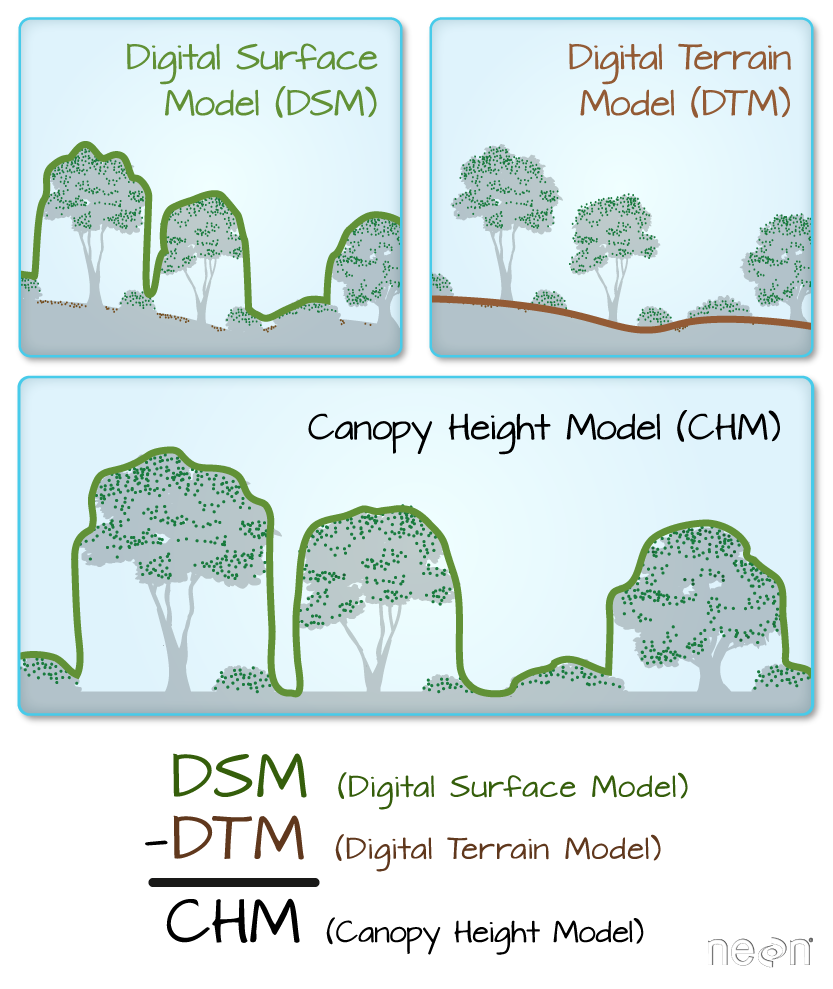## Two Ways to Perform Raster Calculations

We can calculate the difference between two rasters in two different ways:

• by directly subtracting the two rasters in R using raster math

or for more efficient processing - particularly if our rasters are large and/or the calculations we are performing are complex:

• using the `overlay()` function from the `raster` package.

## Raster Math & Canopy Height Models (CHM)

We can perform raster calculations by subtracting (or adding, multiplying, etc) two rasters. In the geospatial world, we call this “raster math”.

Let’s subtract the DTM from the DSM to create a Canopy Height Model. After subtracting, let’s create a dataframe so we can plot with `ggplot`.

``````CHM_HARV <- DSM_HARV - DTM_HARV

CHM_HARV_df <- as.data.frame(CHM_HARV, xy = TRUE)
``````

We can now plot the output CHM.

`````` ggplot() +
geom_raster(data = CHM_HARV_df ,
aes(x = x, y = y, fill = layer)) +
scale_fill_gradientn(name = "Canopy Height", colors = terrain.colors(10)) +
coord_quickmap()
``````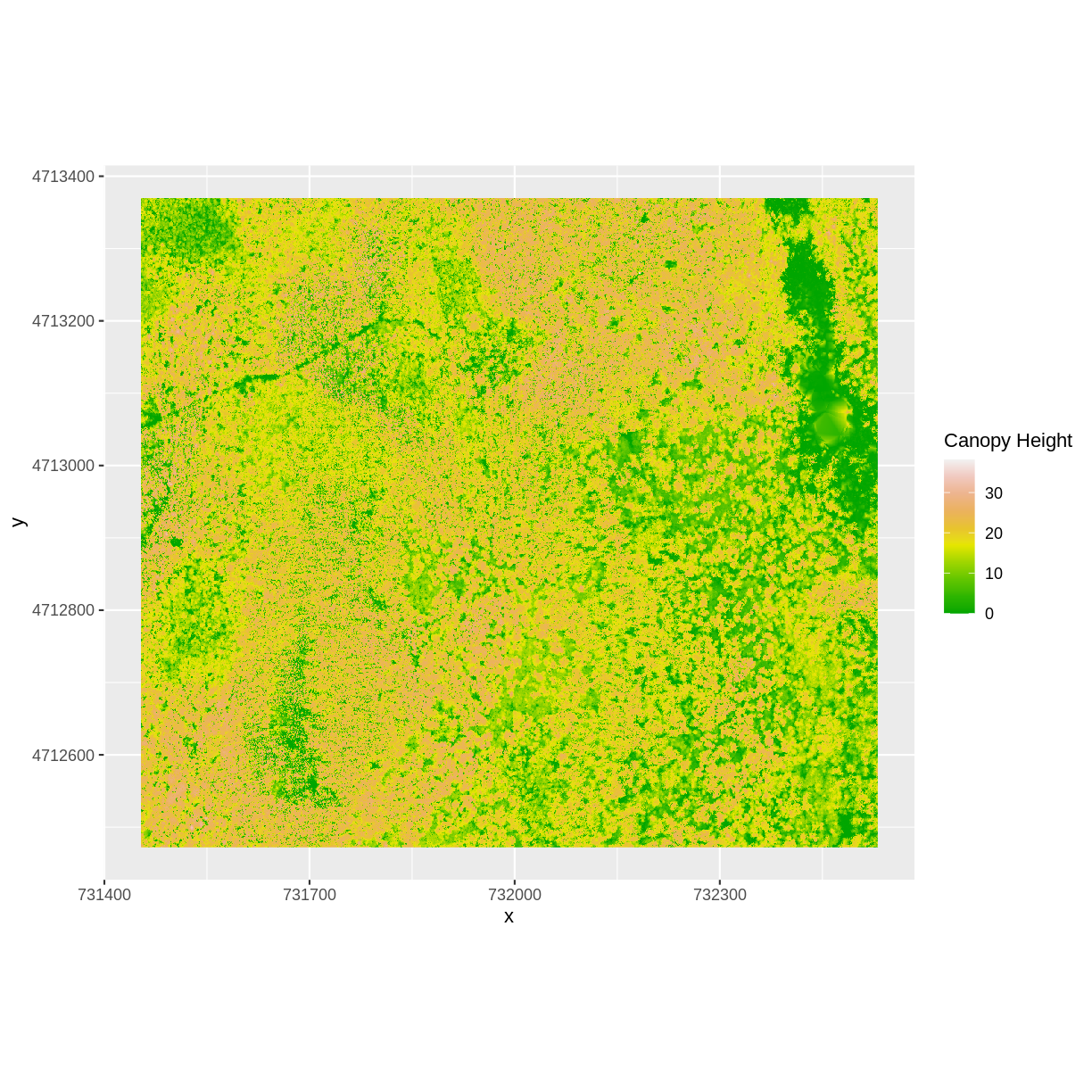Let’s have a look at the distribution of values in our newly created Canopy Height Model (CHM).

``````ggplot(CHM_HARV_df) +
geom_histogram(aes(layer))
``````
```````stat_bin()` using `bins = 30`. Pick better value with `binwidth`.
``````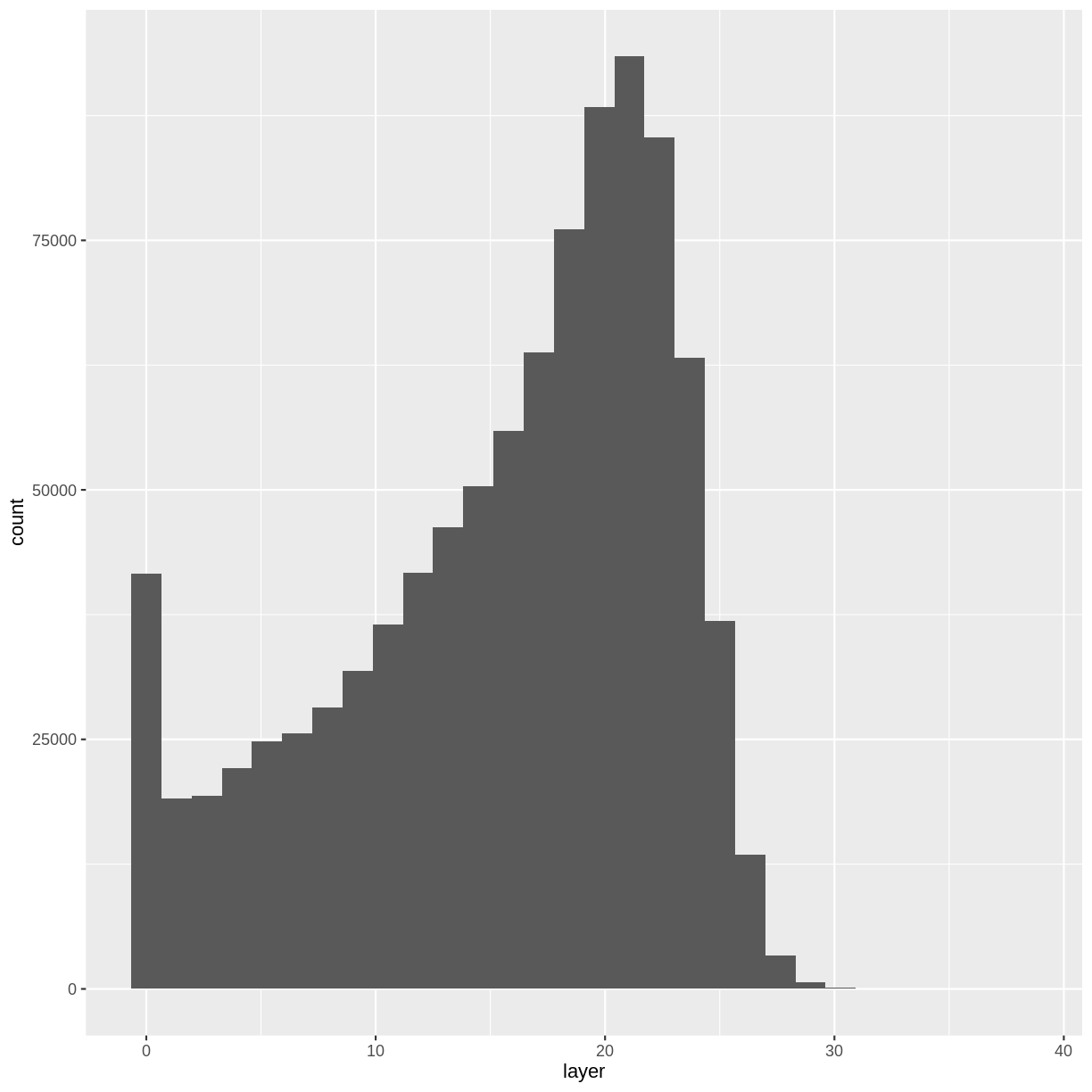Notice that the range of values for the output CHM is between 0 and 30 meters. Does this make sense for trees in Harvard Forest?

## Challenge: Explore CHM Raster Values

It’s often a good idea to explore the range of values in a raster dataset just like we might explore a dataset that we collected in the field.

1. What is the min and maximum value for the Harvard Forest Canopy Height Model (`CHM_HARV`) that we just created?
2. What are two ways you can check this range of data for `CHM_HARV`?
3. Plot a histogram with 6 bins instead of the default and change the color of the histogram.
4. Plot the `CHM_HARV` raster using breaks that make sense for the data. Include an appropriate color palette for the data, plot title and no axes ticks / labels.

## Answers

1) There are missing values in our data, so we need to specify `na.rm = TRUE`.

``````min(CHM_HARV_df\$layer, na.rm = TRUE)
``````
`````` 0
``````
``````max(CHM_HARV_df\$layer, na.rm = TRUE)
``````
`````` 38.16998
``````

2) Possible ways include:

• Create a histogram
• Use the `min()` and `max()` functions.
• Print the object and look at the `values` attribute. 3)
``````ggplot(CHM_HARV_df) +
geom_histogram(aes(layer), colour="black",
fill="darkgreen", bins = 6)
``````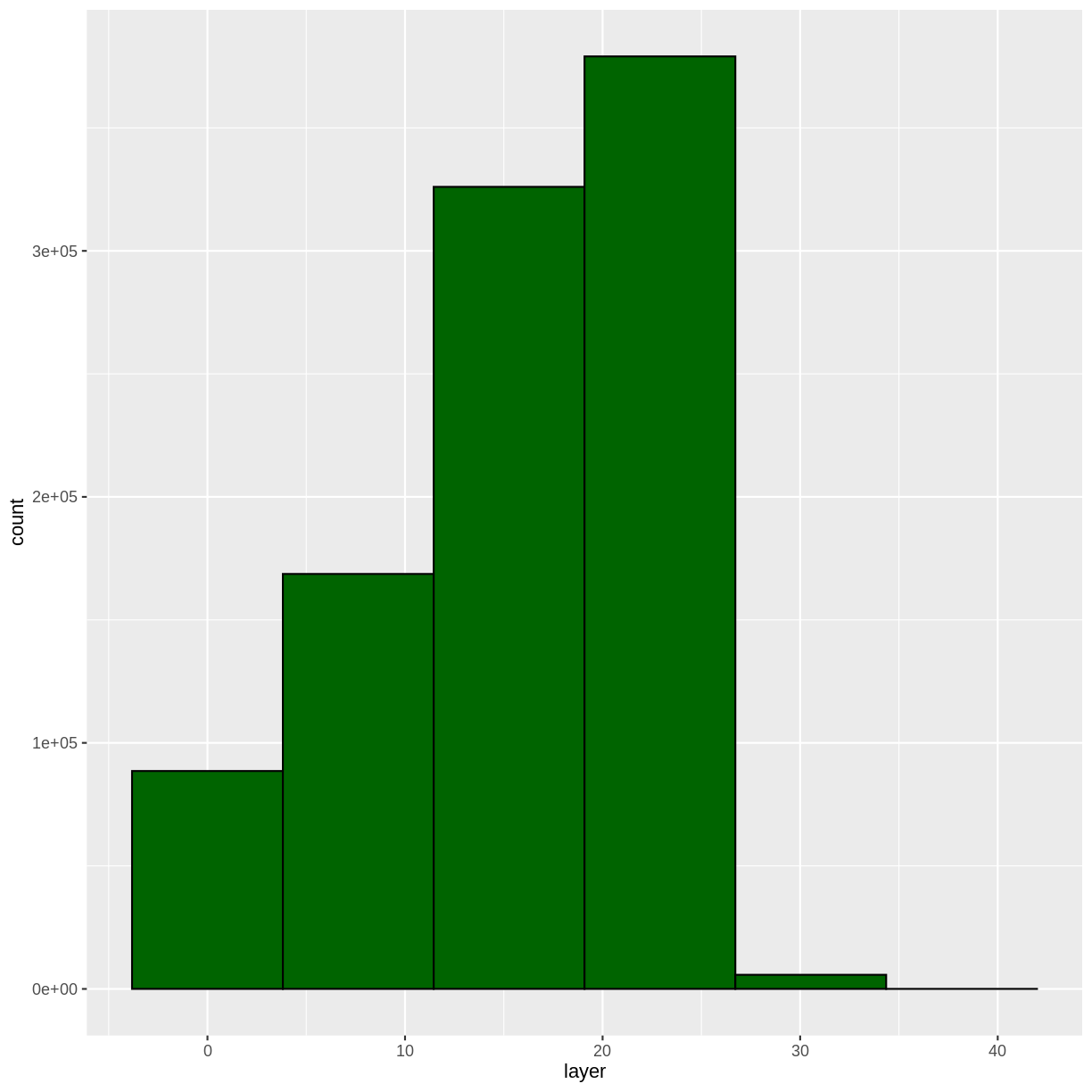4)

``````custom_bins <- c(0, 10, 20, 30, 40)
CHM_HARV_df <- CHM_HARV_df %>%
mutate(canopy_discrete = cut(layer, breaks = custom_bins))

ggplot() +
geom_raster(data = CHM_HARV_df , aes(x = x, y = y,
fill = canopy_discrete)) +
scale_fill_manual(values = terrain.colors(4)) +
coord_quickmap()
``````
``````Warning: Removed 8914 rows containing missing values (geom_raster).
``````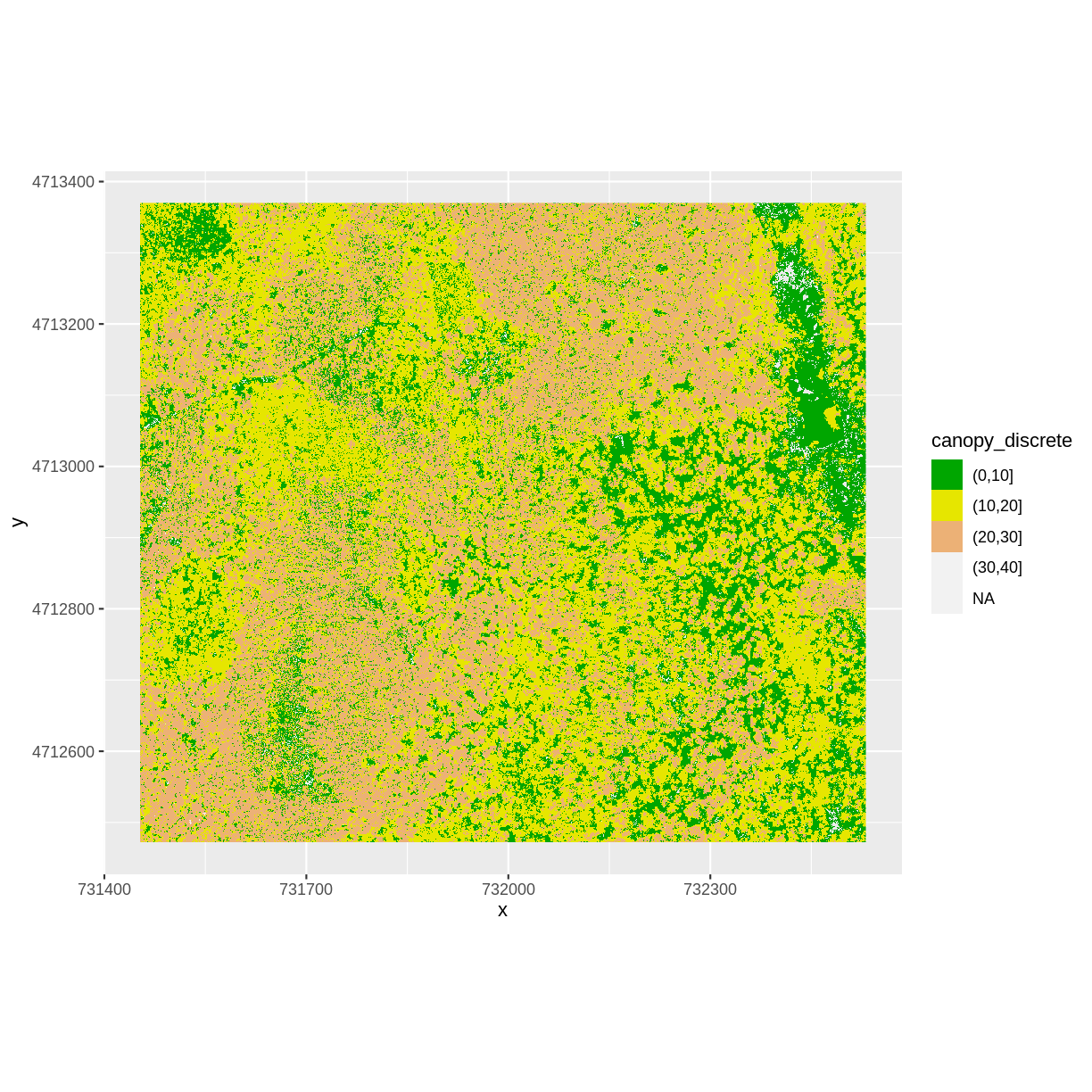## Efficient Raster Calculations: Overlay Function

Raster math, like we just did, is an appropriate approach to raster calculations if:

1. The rasters we are using are small in size.
2. The calculations we are performing are simple.

However, raster math is a less efficient approach as computation becomes more complex or as file sizes become large. The `overlay()` function is more efficient when:

1. The rasters we are using are larger in size.
2. The rasters are stored as individual files.
3. The computations performed are complex.

The `overlay()` function takes two or more rasters and applies a function to them using efficient processing methods. The syntax is

`outputRaster <- overlay(raster1, raster2, fun=functionName)`

## Data Tip

If the rasters are stacked and stored as `RasterStack` or `RasterBrick` objects in R, then we should use `calc()`. `overlay()` will not work on stacked rasters.

Let’s perform the same subtraction calculation that we calculated above using raster math, using the `overlay()` function.

## Data Tip

A custom function consists of a defined set of commands performed on a input object. Custom functions are particularly useful for tasks that need to be repeated over and over in the code. A simplified syntax for writing a custom function in R is: `function_name <- function(variable1, variable2) { WhatYouWantDone, WhatToReturn}`

``````CHM_ov_HARV <- overlay(DSM_HARV,
DTM_HARV,
fun = function(r1, r2) { return( r1 - r2) })
``````
``````CHM_ov_HARV <- overlay(DSM_HARV,
DTM_HARV,
fun = function(r1, r2) { return( r1 - r2) })
``````

Next we need to convert our new object to a data frame for plotting with `ggplot`.

``````CHM_ov_HARV_df <- as.data.frame(CHM_ov_HARV, xy = TRUE)
``````

Now we can plot the CHM:

`````` ggplot() +
geom_raster(data = CHM_ov_HARV_df,
aes(x = x, y = y, fill = layer)) +
scale_fill_gradientn(name = "Canopy Height", colors = terrain.colors(10)) +
coord_quickmap()
``````How do the plots of the CHM created with manual raster math and the `overlay()` function compare?

## Export a GeoTIFF

Now that we’ve created a new raster, let’s export the data as a GeoTIFF file using the `writeRaster()` function.

When we write this raster object to a GeoTIFF file we’ll name it `CHM_HARV.tiff`. This name allows us to quickly remember both what the data contains (CHM data) and for where (HARVard Forest). The `writeRaster()` function by default writes the output file to your working directory unless you specify a full file path.

We will specify the output format (“GTiff”), the no data value (`NAflag = -9999`. We will also tell R to overwrite any data that is already in a file of the same name.

``````writeRaster(CHM_ov_HARV, "CHM_HARV.tiff",
format="GTiff",
overwrite=TRUE,
NAflag=-9999)
``````

### writeRaster() Options

The function arguments that we used above include:

• format: specify that the format will be `GTiff` or GeoTIFF.
• overwrite: If TRUE, R will overwrite any existing file with the same name in the specified directory. USE THIS SETTING WITH CAUTION!
• NAflag: set the GeoTIFF tag for `NoDataValue` to -9999, the National Ecological Observatory Network’s (NEON) standard `NoDataValue`.

## Challenge: Explore the NEON San Joaquin Experimental Range Field Site

Data are often more interesting and powerful when we compare them across various locations. Let’s compare some data collected over Harvard Forest to data collected in Southern California. The NEON San Joaquin Experimental Range (SJER) field site located in Southern California has a very different ecosystem and climate than the NEON Harvard Forest Field Site in Massachusetts.

Import the SJER DSM and DTM raster files and create a Canopy Height Model. Then compare the two sites. Be sure to name your R objects and outputs carefully, as follows: objectType_SJER (e.g. `DSM_SJER`). This will help you keep track of data from different sites!

1. You should have the DSM and DTM data for the SJER site already loaded from the Plot Raster Data in R episode.) Don’t forget to check the CRSs and units of the data.
2. Create a CHM from the two raster layers and check to make sure the data are what you expect.
3. Plot the CHM from SJER.

## Answers

1) Use the `overlay()` function to subtract the two rasters & create the CHM.

``````CHM_ov_SJER <- overlay(DSM_SJER,
DTM_SJER,
fun = function(r1, r2){ return(r1 - r2) })
``````

Convert the output to a dataframe:

``````CHM_ov_SJER_df <- as.data.frame(CHM_ov_SJER, xy = TRUE)
``````

Create a histogram to check that the data distribution makes sense:

``````ggplot(CHM_ov_SJER_df) +
geom_histogram(aes(layer))
``````
```````stat_bin()` using `bins = 30`. Pick better value with `binwidth`.
``````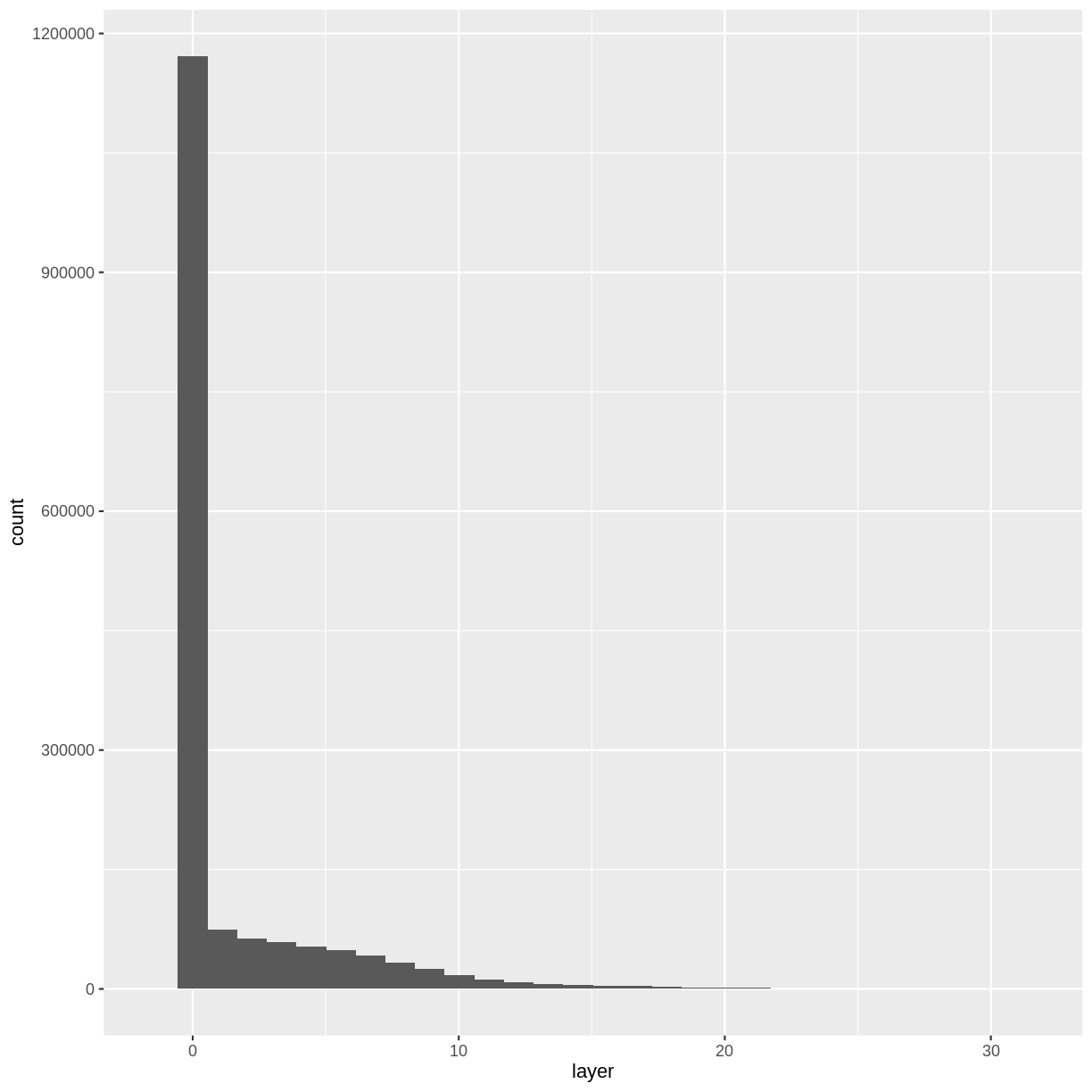2) Create a plot of the CHM:

`````` ggplot() +
geom_raster(data = CHM_ov_SJER_df,
aes(x = x, y = y,
fill = layer)
) +
scale_fill_gradientn(name = "Canopy Height",
colors = terrain.colors(10)) +
coord_quickmap()
``````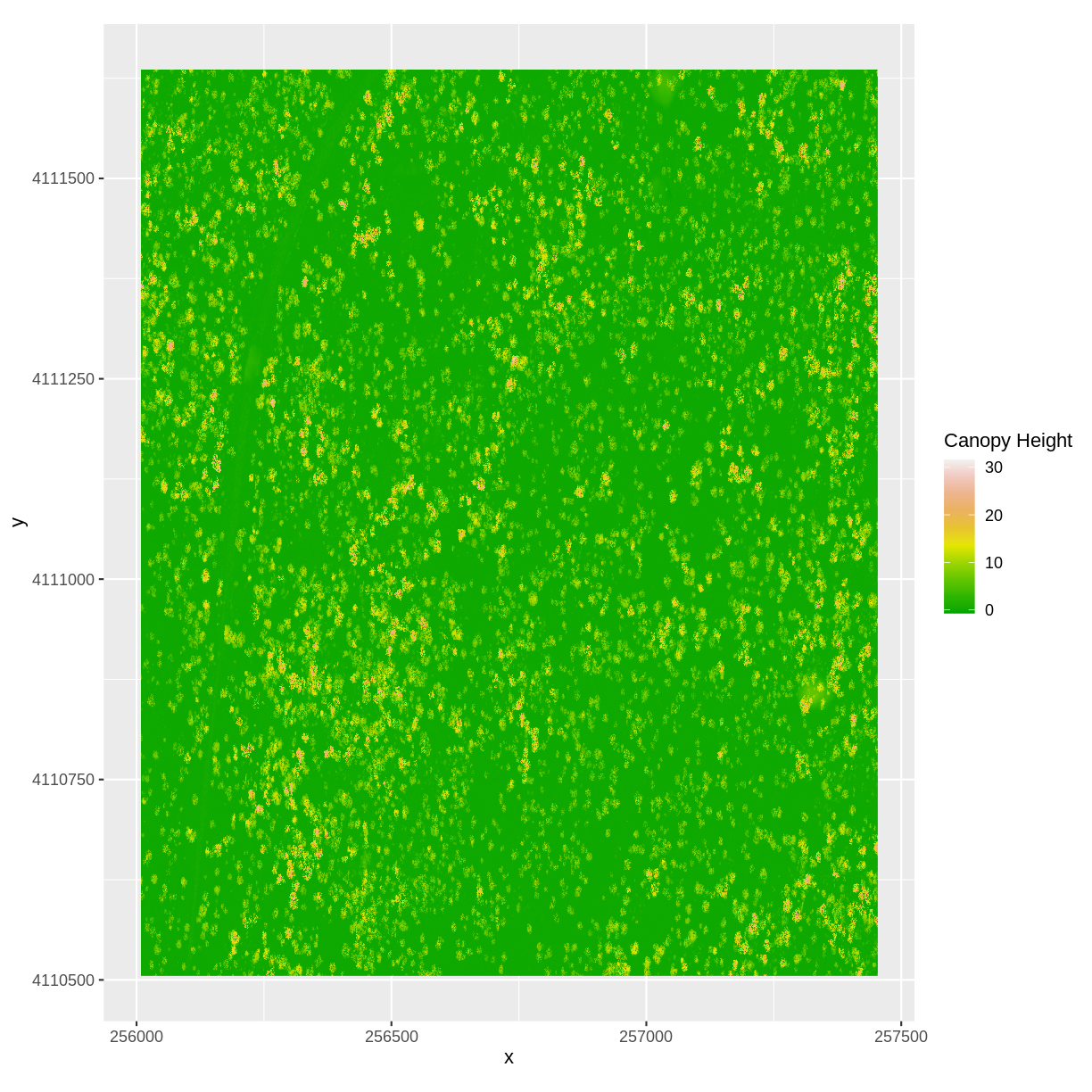## Key Points

• Rasters can be computed on using mathematical functions.

• The `overlay()` function provides an efficient way to do raster math.

• The `writeRaster()` function can be used to write raster data to a file.